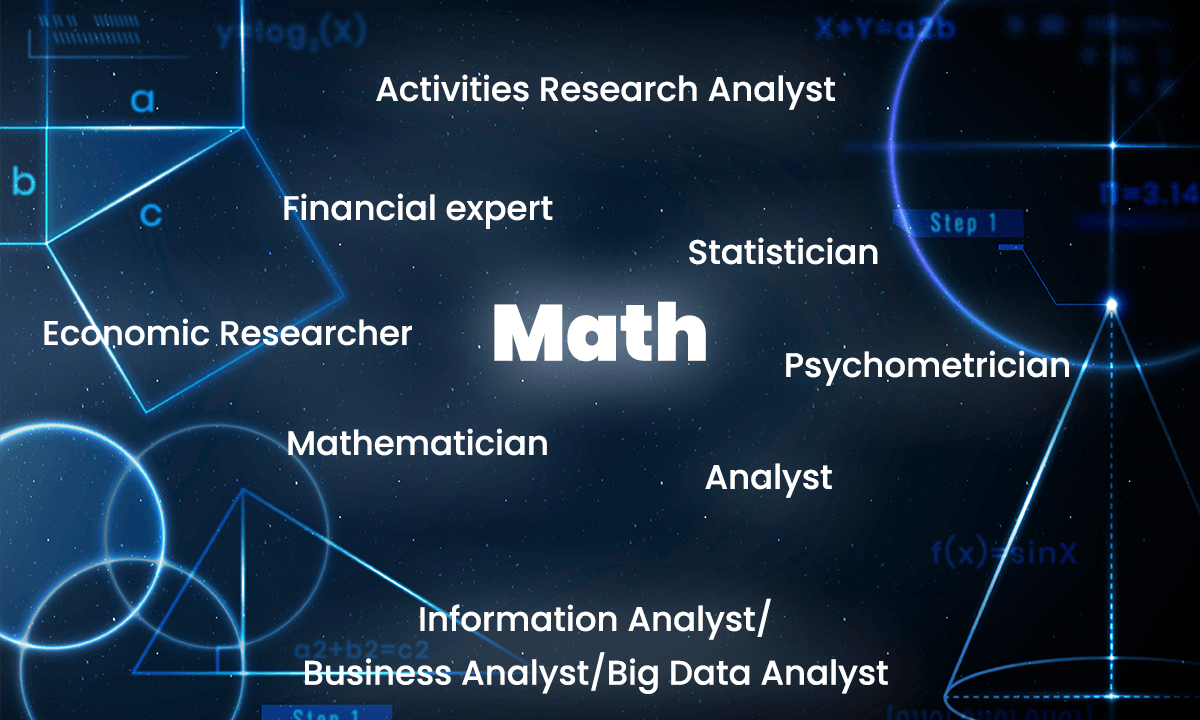Online math technology Certification Courses - Learn Anytime, Anywhere | EasyShiksha

# Math

Maths is the study of design, request, and connection that has advanced from natural acts of tallying, estimating, and portraying the states of articles. It manages legitimate thinking and quantitative computation, and its advancement has included an expanding level of glorification and deliberation of its topic. Since the seventeenth century, math has been an essential subordinate to the actual sciences and innovation, and on later occasions, it plays a comparable part in the quantitative parts of the existing sciences.

In many societies—under the improvement of the requirements of common sense pursuits, like business and horticulture—math has created a long way past fundamental tallying. This development has been most noteworthy in social orders sufficiently complex to support these exercises and to give relaxation to examination and the chance to expand on the accomplishments of prior mathematicians. Algebra, number theory, geometry, and arithmetic are the four main fields of mathematics.

Arithmetic: Among the various fields of mathematics, it is the oldest and most fundamental. It is concerned with numbers and their basic operations, such as addition, subtraction, multiplication, and division.

Algebra: It's a type of arithmetic in which we combine integers with unknown quantities. The letters of the English alphabet, such as X, Y, A, B, and so on, or symbols, are used to represent these unknown numbers. Letters aid in the generalisation of formulas and principles, as well as the identification of unknown missing values in algebraic expressions and equations.

Geometry: It is the branch of mathematics that deals with the preparation of figures' shapes and sizes, as well as their qualities. Points, lines, angles, surfaces, and solids are the basic constituents of geometry.

Trigonometry: It is the study of relationships between angles and sides of triangles, which is derived from two Greek concepts, trigon (meaning triangle) and metron (means measurement).

Analysis: It is the branch of mathematics that studies the rate of change in various quantities. Calculus is the foundation of analysis.

The following are the jobs available for them• Analyst
• Mathematician
• Activities Research Analyst﻿
• Statistician
• Information Analyst/Business Analyst/Big Data Analyst
• Financial expert
• Economic Researcher
• Psychometrician